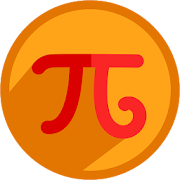# Math Formulas Free - Lumos Educational App Store4.61
Price -FREE
\$0

#### DESCRIPTION:

Math Formulas aggregated in the most useful way. The App covers following topics 1) Geometry 2) Algebra 3) Trigonometry 4) Equations 5) Analytic Geometry 6) Derivative 7) Integration 8) Matrices 9) Statistics 10) Units Conversion 11) Math Tricks 12) Favorite 13) Tool 13.1 Units Conversion 13.2 2D Shapes Calculator 13.3 3D Shapes Calculator 13.4 Equation Solver 13.5 Analytic Geometry Please email us at to add any new functions or suggestions.

#### OVERVIEW:

Math Formulas Free is a free educational mobile app By .It helps students in grades HS practice the following standards HSN.Q.A.1.

This page not only allows students and teachers download Math Formulas Free but also find engaging Sample Questions, Videos, Pins, Worksheets, Books related to the following topics.

1. HSN.Q.A.1 : Use units as a way to understand problems and to guide the solution of multi-step problems; choose and interpret units consistently in formulas; choose and interpret the scale and the origin in graphs and data displays..

HS

#### STANDARDS:

HSN.Q.A.1

Developer:

Software Version: 1.0.2

Category: Education

### RELATED APPSEdSearch WebSearch